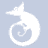quizzes  Forums

# Design Of Experiments

1 508 played - 11 yrs ago
Questions about what we've learned so far.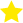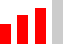30 QUESTIONS
51%1
We can use use ANOVA to test the equality of two or more means.

2
What is meant by a replication of an experiment?

3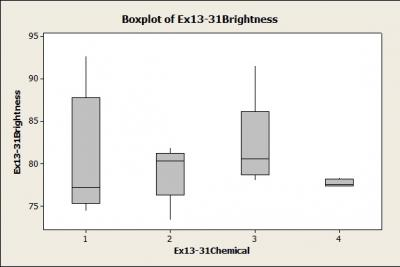Does the picture below suggest that we have a significant result for differences between treatments.

4
In a completely randomized experiment all of the runs are made in random order.

5
Why randomize the application of treatments in the first place?

6
In a fixed effect experiments, the treatments are chosen at random.

7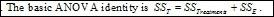The basic anova identity is shown below.

8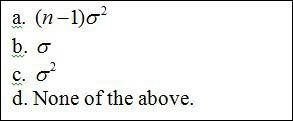Which is the correct value for the expected value of the mean square of the error?

9
If the F-statistic in a single-factor ANOVA is significant this indicates that all of the means being compared are different.

10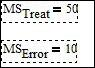According to the data given below, what is the value of the F statistic?

11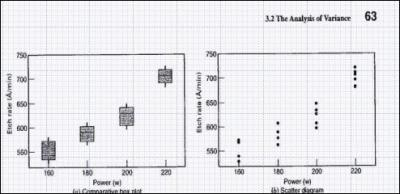In the picture below, does it seem that the results of the should be significant or not? That is, does it seem that at least one of the treatment means is different from the others?

12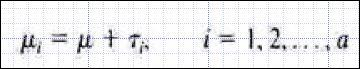In the single-factor ANOVA model, the mean of treatment i is the sum of the grand mean and the ith treatment effect. Is the picture below correct?

13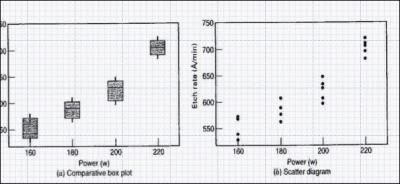In the picture below, what exactly is the factor and what are the treatment levels and the response?

14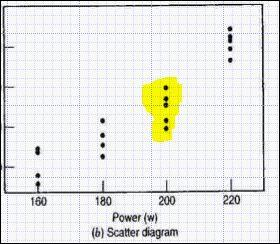In the picture below, we see one one replicate of the experiment highlited.

15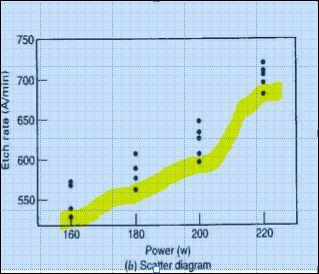In the picture below, we see one full replicate of the experiment highlited

16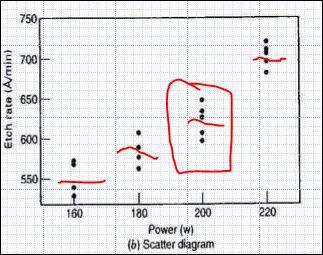In the picture, how many runs are in the red box? How many deviations from this treatment mean? How many degrees of freedom for error under this treatment?

17
In the picture on the left, we see 3 runs under level 1 of the factor. This means there is a mean and consequently 2 degrees of freedom for error with respect to this mean. We have 4 all together.

18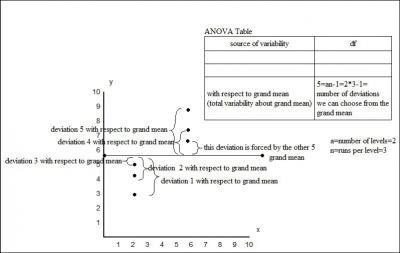In the picture we see 6 data points, and 1 grand mean. This means we can calculate 5 deviations from this mean the 6 is forced by the values of the first 5. So we have only 5 degrees of freedom.

19
Is the picture shown on the left correct or incorrent?

20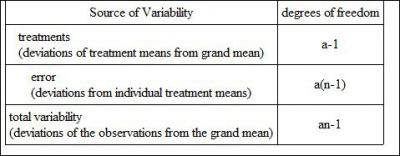In the table below, if I increase n, what happens to the number of dots in a scatter diagram at every treatment level? Do the dots increase in number vertically or horizontally?

21If I increase a in the table below, what will happen to the number of dots in a scatter diagram? Will the increase come from adding dots vertically at every level or horizontally WITH new levels?

22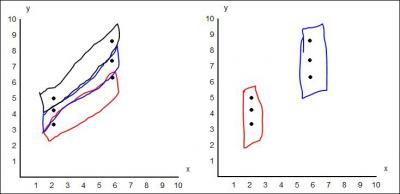Which picture, the left or right, shows the correct groupings in terms of the definition of a replicate of an experiment?

23
If in the table below, I increase n, will the degrees of freedom for error increase or decrease? Is the total variabiilty in the data affected? Are the degrees of freedom for treatments affected?

24
In the table below, if we change the number of dots in the scatter diagram per level from let's, say, 3 to 5, which of the following will happen?

25
If in the picture below, I change the number of levels from, let's say 2 to 3, what will happen ?

26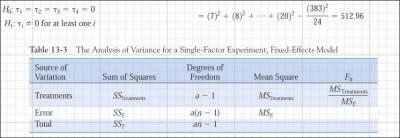Below is a correctly completed table for one factor randomized experiment.

27Below is a correctly completed table for a one factor randomized experiment with blocking.

28
Why is repeated measures given the name it's given?

29
If we have 2 factors and two levels per factors, what kind of experiment is this?

30
If we have 2^3 experiment, what does this mean and we run 10 replicates, what's the result?